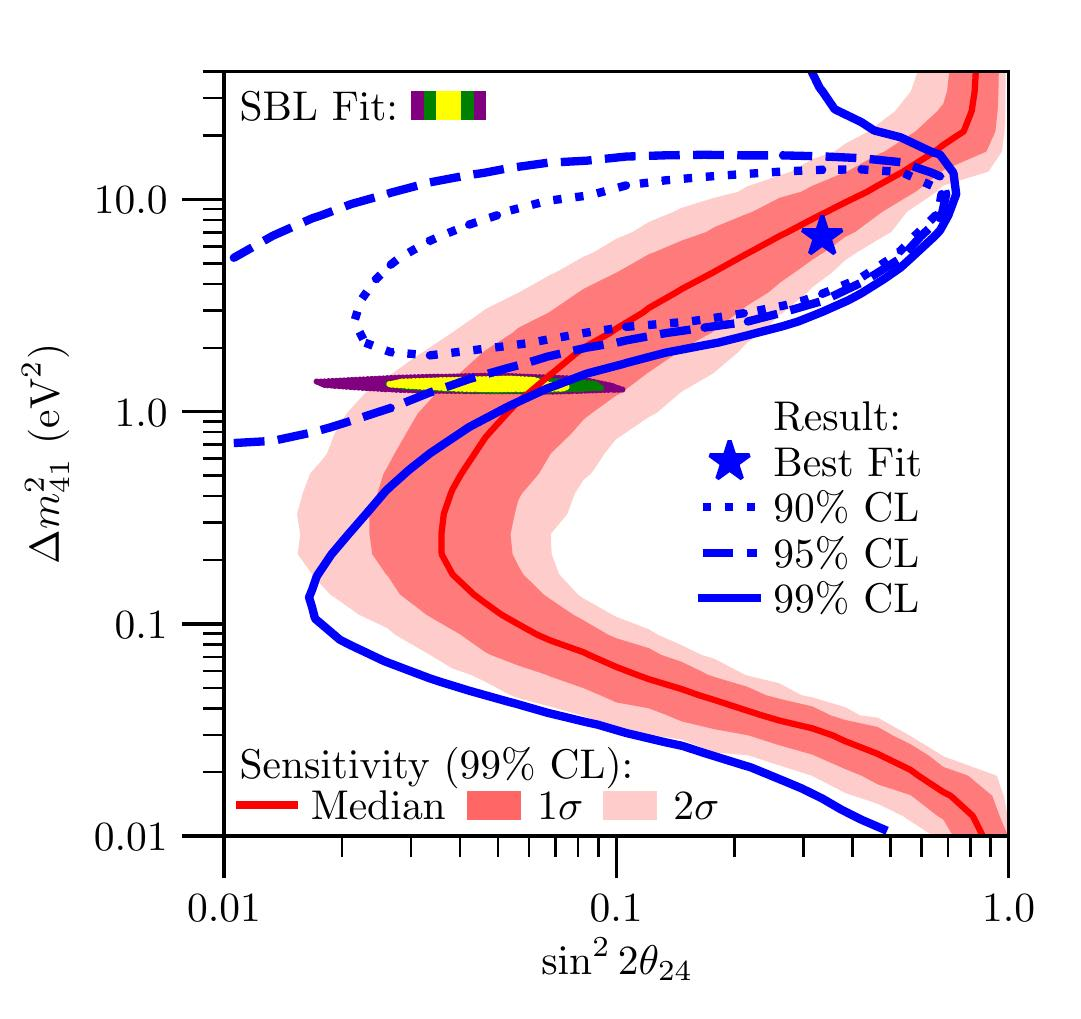# IceCube conducts innovative search for unstable sterile neutrinos

For over 20 years, physicists have performed experiments that hint at the existence of an elusive fourth type of neutrino, the “sterile” neutrino. Neutrinos—tiny, almost massless particles—are also known as “ghost particles” because they rarely interact with the matter they travel through. On the other hand, the appropriately named sterile neutrino does not interact with matter at all.If sterile neutrinos exist, IceCube would measure the disappearance of atmospheric neutrinos that would otherwise reach the detector on a trajectory through the Earth’s core. Credit: IceCube Collaboration.

The notion of a sterile neutrino challenges the Standard Model of particle physics where neutrinos come in three flavors: muon, electron, and tau. In a “3+1” model, a fourth, heavier neutrino mass state is considered along with the three known mass states. This fourth neutrino mass state consists mostly of the sterile flavor. Now, IceCube researchers are considering another model, known as the “3+1+decay” or unstable sterile neutrino model, where the unstable fourth mass state can decay into lighter invisible particles.

In a new study accepted today by Physical Review Letters, the IceCube Collaboration presents its first search for the unstable sterile neutrino. Although IceCube placed strong constraints on the existence of a sterile neutrino in 2016, anomalous results in accelerator neutrino experiments still seem to favor a fourth noninteracting neutrino flavor. In order to reconcile the constraints and results, physicists have been exploring extensions to the “3+1” model. In the Standard Model, particles that are not protected by a fundamental symmetry decay. This motivated scientists to also consider the unstable sterile neutrino model. For the present study, the unstable sterile neutrino model was fitted to eight years of data collected from the IceCube Neutrino Observatory, a neutrino detector with sensor arrays buried beneath the South Pole ice.

Since sterile neutrinos cannot be observed directly, the researchers looked at the effects of sterile neutrinos on the detection rates of another neutrino flavor, the muon neutrino. When a muon neutrino crashes into a nucleus, a muon is created, along with a streak of blue Cherenkov light that is picked up by the light-detecting sensors in IceCube. Due to a phenomenon called neutrino oscillation—where a neutrino can convert into another neutrino flavor when hurtling through space, researchers focused on deficits in the number of detected muon neutrinos for hints of sterile neutrinos.

The analysis was led by IceCube collaborator Marjon Moulai, a postdoctoral Balzan Fellow at the Wisconsin IceCube Particle Astrophysics Center at the University of Wisconsin–Madison. “We caught muons that are produced when muon neutrinos interact with matter since we can’t detect muon neutrinos directly,” explained Moulai, who conducted most of the work as a PhD student at the Massachusetts Institute of Technology. “Because muons cannot travel far through the Earth, while neutrinos can, we know that these muons were produced by muon-neutrino interactions.”

The researchers collected a large sample of muons that originated from these muon-neutrino interactions and measured their disappearance probabilities. The analysis zeroed in on muons with energies between 500 GeV and 10 TeV. To calculate muon disappearance probabilities, the researchers estimated the zenith angles and energies of the muons. They then calculated the consequence of the unstable sterile neutrino model on the muon neutrino distribution of energy and zenith angle. The expected distributions were finally compared to the data sample.Results from the first IceCube search. The blue curves are the frequentist allowed regions for g2 = 2.5π, which is the best-fit decay coupling. The best-fit point is shown with a blue star. The sensitivity bands are shown in pink. The allowed regions from complementary short-baseline experiments are the yellow/green/purple islands. Credit: IceCube Collaboration.

“We found that the unstable sterile neutrino model fits the data better than the standard model of only three neutrinos, and that this model fits the data better than the traditional 3+1 model,” said Moulai. Moulai further concluded that although both the standard three neutrino model (p-value = 2.8%) and the 3+1 model (p-value = 0.81%) were disfavored, the evidence favoring an unstable sterile neutrino is not statistically significant.

“We must follow the hints we have found and test this hypothesis further,” said Moulai. “There are other ongoing searches for sterile neutrinos within and beyond IceCube.”

Currently, IceCube researchers are harnessing more advanced tools that can better reconstruct energies for studying muon neutrinos. Future studies may also look at the effect of sterile neutrinos on the distribution of tau neutrinos, one of the other neutrino flavors. With these new hints and future advances, the puzzle of the elusive fourth neutrino will only become clearer.

+ info “Search for Unstable Sterile Neutrinos with the IceCube Neutrino Observatory,” IceCube Collaboration: R. Abbasi et al.. Physical Review Letters 129 (2022) 15, 15, journals.aps.org, https://arxiv.org/abs/2204.00612